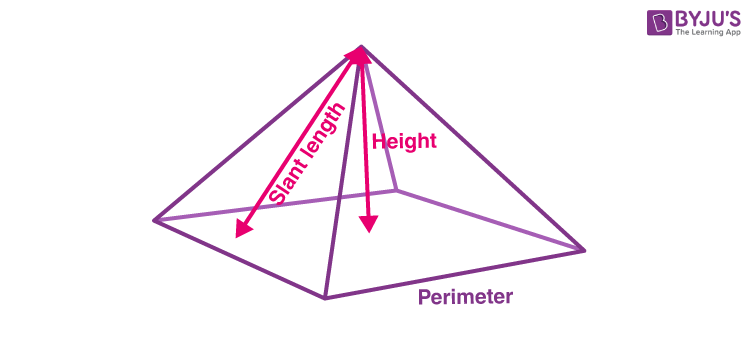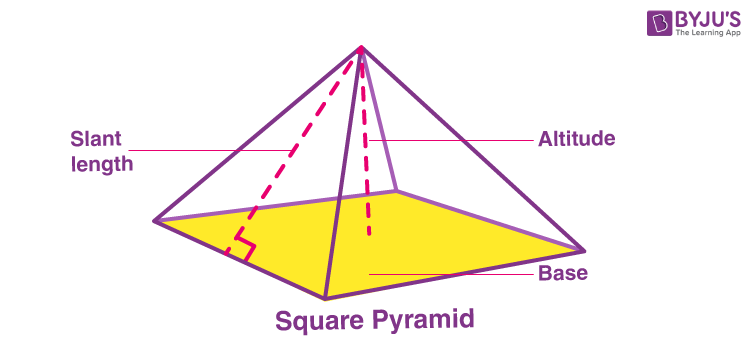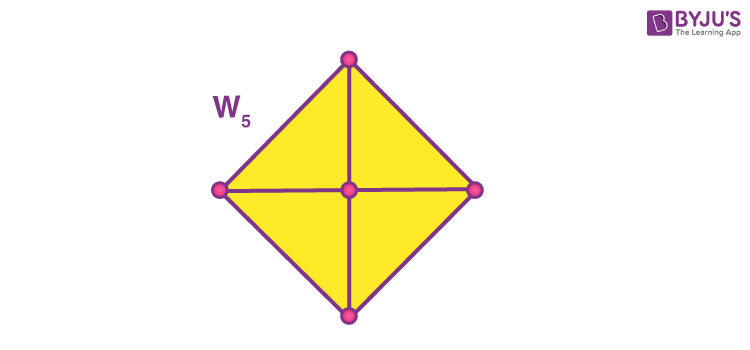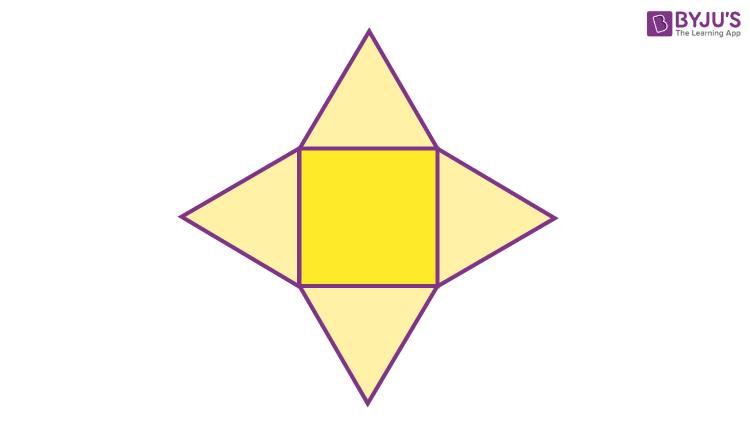# Square Pyramid

A Pyramid is a polyhedron that has a base and 3 or greater triangular faces that meet at a point above the base (the apex). In the case of a square pyramid, the base is in square shape and it has four faces. A most famous example of such a pyramid in real life is the Great Pyramid of Giza. On a small scale when we learn about pyramids, then we have to learn the other concepts related to them, such as volume of pyramid, area of the pyramid. In this article, we will learn the definition of a square pyramid, types, properties, area and volume formulas with many solved examples.## Square Pyramid Definition

A three-dimensional geometric shape having a square base and four triangular faces/sides that meet at a single point is called a square pyramid. If all the triangular faces have equal edges, then this pyramid is said to be an equilateral square pyramid. If the apex of the pyramid is right above the centre of its base, it forms a perpendicular with the base and such a square pyramid is known as the right square pyramid.## Representation of Square Pyramid

A square pyramid is shown by the Wheel graph W5. A wheel graph is one formed by connecting a single universal vertex to all vertices of a cycle.## Types of Square Pyramid

The different types of square pyramids are :

• Equilateral Square Pyramid
• Right Square Pyramid

### Equilateral Square Pyramid

If all the edges are of equal length, then the sides form equilateral triangles, and the pyramid is an equilateral square pyramid.

The Johnson square pyramid can be classified by a single edge-length parameter l. The height h, the surface area A, and the volume V of such a pyramid are:

• h = (1/√2)l
• A = (1+√3)l2
• V = (√2/6)l3

### Right Square Pyramid

In a right square pyramid, all the lateral edges are of the same length, and the sides other than the base are congruent isosceles triangles.

A right square pyramid with base length l and height h has the following formula for surface area and volume:

• $$Area=l^{2}+l\sqrt{l^{2}+(2h)^{2}}$$
• Volume=(⅓)×l2×h
• $$Lateral\ edge\ length =\sqrt{h^{2}+\frac{l^{2}}{2}}$$
• $$Slant\ Height = \sqrt{h^{2}+\frac{l^{2}}{4}}$$

## Properties of Square Pyramid

• It has 5 Faces
• The 4 Side Faces are Triangles
• The Base is a Square
• It has 5 Vertices (corner points)
• It has 8 Edges

## Square Pyramid Formula

There are formulas for square pyramid based on volume, area, height and surface area.

### The Volume of Square Pyramid

$$V = a^{2}\frac{h}{3}$$

Where a is the base edge length.

h is the height.

### The Surface Area of a Square Pyramid

$$A = a^{2}+2a\sqrt{\frac{a^{2}}{4}+h^{2}}$$

Where a is the base edge length.

h is the height.

The general formula for the total surface area of a regular pyramid is given by:

T.S.A.= ½ Pl + B

where,

P is the perimeter of the base

l the slant height and

B the area of the base

### Lateral Edge Length

$$e=\sqrt{h^{2}+\frac{l^{2}}{2}}$$

Where h is the height

And l is the base length

### Height of square pyramid

$$e=\sqrt{h^{2}+\frac{l^{2}}{4}}$$

Where h is the height

And l is the base length

## Net of a Square Pyramid

A net of a 3D shape is a pattern reached when the surface of a three-dimensional figure is laid out horizontally showing each face of the figure. A solid may have different nets.

Steps to determine whether net forms a solid are as follows:

1. Solid and the net should have the same number of faces. Also, the shapes of the faces of the solid should match with the shapes of the corresponding faces in the net.
2. Imagine how the net is to be folded to create the solid and assure that all the sides fit collectively properly.

Nets are of use when we need to find the surface area of the solids.### Square Pyramid Examples

Example 1:

Find the volume of a regular square pyramid with base sides 10 cm and altitude 18 cm.

Solution:

The formula for the volume of a pyramid is given by:

V = ⅓ B h

As the base of the pyramid is a square, the base area is 102 or 100  cm2.

So, put 100 for B and 18 for h in the formula.

V=13×100×18=600

Therefore, the volume of the given square pyramid is 600  cm3.

Example 2:

Find the lateral surface area of a regular pyramid that has a triangular base and each edge of the base is equal to 8 cms and the slant height is 5 cms.

Solution:

The perimeter of the base is the sum of the sides.

Perimeter = 3 × 8 = 24  cms

L.S.A.=12 × 24 × 5 = 60  cms2

Example 3:

Find the total surface area of a regular pyramid having a square base where the length of each edge of the base is equal to 16 cms, the slant height of a side is 17 cms and the altitude is 15 cms.

Solution:

The perimeter of the base is 4s because it is a square.

P = 4 × 16 = 64 cms

The area of the square base = s2

Base area = 162 = 256  cms2

Therefore, the total surface area of the pyramid,

T.S.A.=½ × 64 × 17 + 256  = 544 + 256  = 800  cms2

## Frequently Asked Questions on Square Pyramid

### What is a square pyramid?

A square pyramid is a three-dimensional figure with a square base and four triangular faces joined at a vertex.

### Mention the few properties of a square pyramid?

A square pyramid has a square base.
It has four triangular faces and 5 vertices.
It has 8 edges.

### What is the formula for the volume of a square pyramid?

The formula for square pyramid is (⅓)(Base area of a square)(Height of the square pyramid) cubic units.

### What is the total surface area of a square pyramid?

The total surface area of a square pyramid is (½)Pl + B
Where “P” is the perimeter of the square pyramid
“l” is the slant height
“B” is the base area of a square.

### What is the right square pyramid?

If the apex of the square pyramid is perpendicularly above the centre of the square, then it is called the right square pyramid.

Quiz on Square Pyramid

#### 1 Comment

1. Arya Keshri

The volume and surface area of a pyramid with a square base of area 𝑎
2
and height h is
given by 𝑉 =
ℎ𝑎
2
3
and 𝑆 = 𝑎
2 + 2𝑎√(𝑎/2)
2 + ℎ
2.